# Meta analysis

4 de Mar de 2020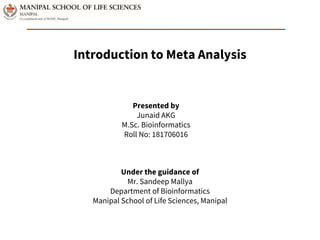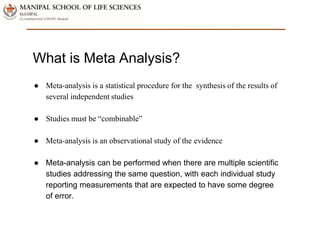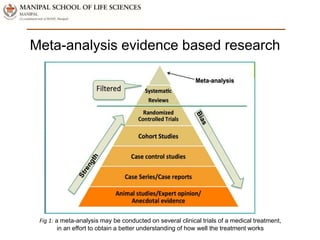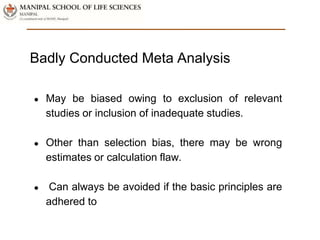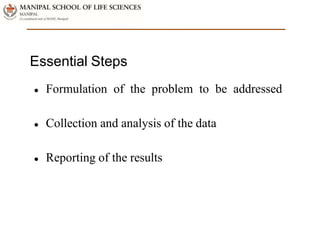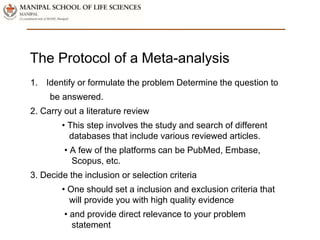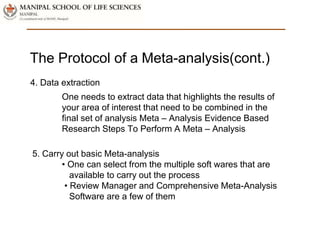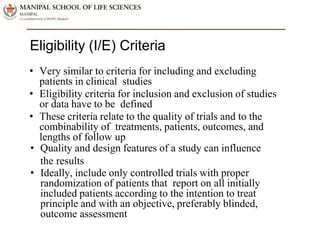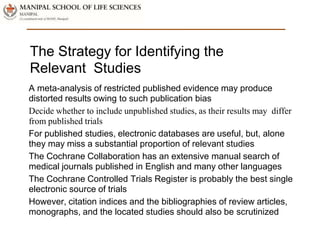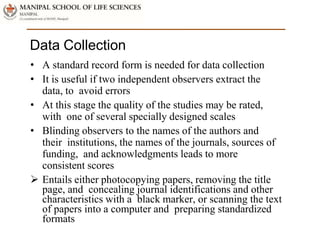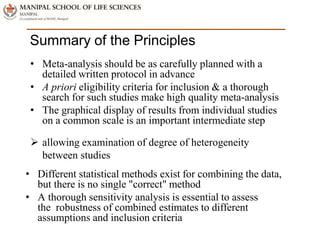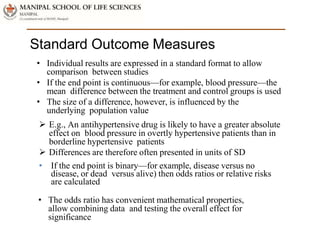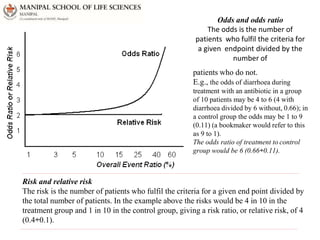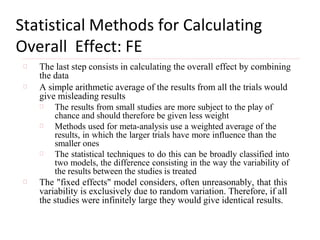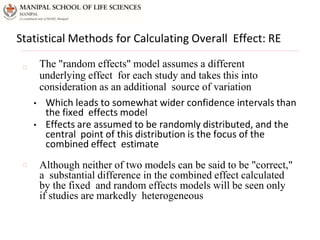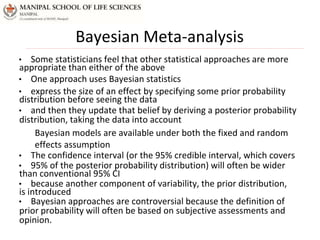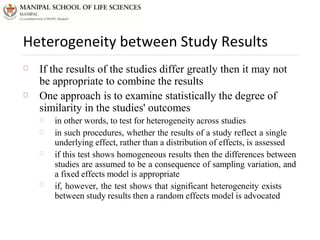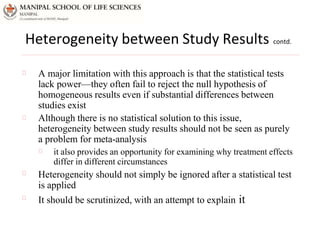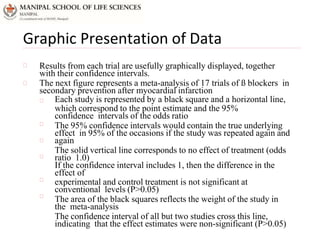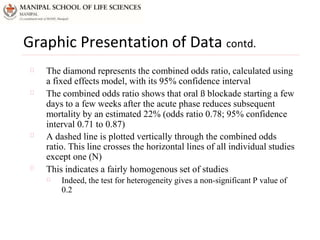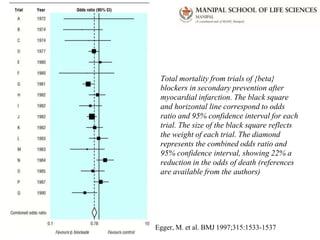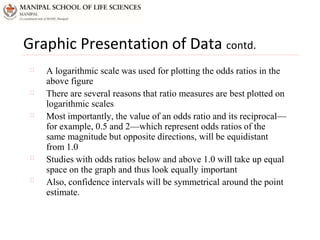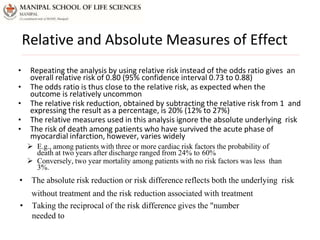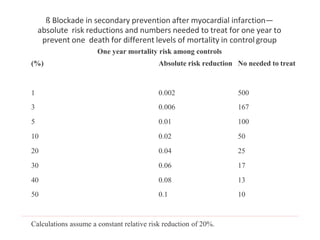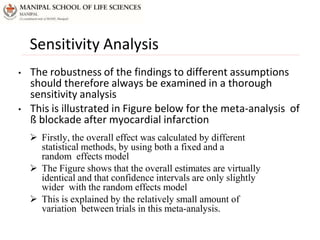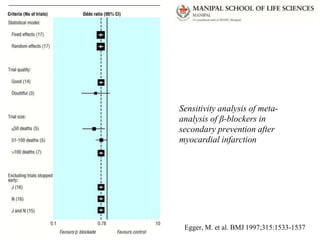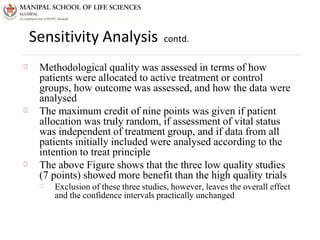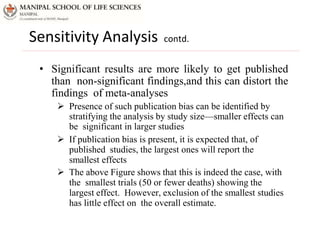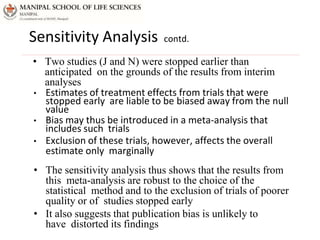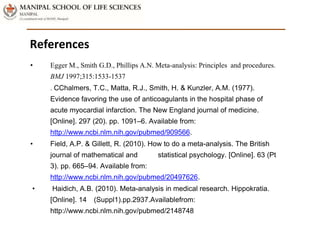1 de 32

### Meta analysis

• 1. Introduction to Meta Analysis Presented by Junaid AKG M.Sc. Bioinformatics Roll No: 181706016 Under the guidance of Mr. Sandeep Mallya Department of Bioinformatics Manipal School of Life Sciences, Manipal
• 2. What is Meta Analysis? ● Meta-analysis is a statistical procedure for the synthesis of the results of several independent studies ● Studies must be “combinable” ● Meta-analysis is an observational study of the evidence ● Meta-analysis can be performed when there are multiple scientific studies addressing the same question, with each individual study reporting measurements that are expected to have some degree of error.
• 3. Meta-analysis evidence based research Fig 1: a meta-analysis may be conducted on several clinical trials of a medical treatment, in an effort to obtain a better understanding of how well the treatment works
• 4. ● May be biased owing to exclusion of relevant studies or inclusion of inadequate studies. ● Other than selection bias, there may be wrong estimates or calculation flaw. ● Can always be avoided if the basic principles are adhered to Badly Conducted Meta Analysis
• 5. Essential Steps ● Formulation of the problem to be addressed ● Collection and analysis of the data ● Reporting of the results
• 6. The Protocol of a Meta-analysis 1. Identify or formulate the problem Determine the question to be answered. 2. Carry out a literature review • This step involves the study and search of different databases that include various reviewed articles. • A few of the platforms can be PubMed, Embase, Scopus, etc. 3. Decide the inclusion or selection criteria • One should set a inclusion and exclusion criteria that will provide you with high quality evidence • and provide direct relevance to your problem statement
• 7. The Protocol of a Meta-analysis(cont.) 4. Data extraction One needs to extract data that highlights the results of your area of interest that need to be combined in the final set of analysis Meta – Analysis Evidence Based Research Steps To Perform A Meta – Analysis 5. Carry out basic Meta-analysis • One can select from the multiple soft wares that are available to carry out the process • Review Manager and Comprehensive Meta-Analysis Software are a few of them
• 8. Eligibility (I/E) Criteria • Very similar to criteria for including and excluding patients in clinical studies • Eligibility criteria for inclusion and exclusion of studies or data have to be defined • These criteria relate to the quality of trials and to the combinability of treatments, patients, outcomes, and lengths of follow up • Quality and design features of a study can influence the results • Ideally, include only controlled trials with proper randomization of patients that report on all initially included patients according to the intention to treat principle and with an objective, preferably blinded, outcome assessment
• 9. The Strategy for Identifying the Relevant Studies A meta-analysis of restricted published evidence may produce distorted results owing to such publication bias Decide whether to include unpublished studies, as their results may differ from published trials For published studies, electronic databases are useful, but, alone they may miss a substantial proportion of relevant studies The Cochrane Collaboration has an extensive manual search of medical journals published in English and many other languages The Cochrane Controlled Trials Register is probably the best single electronic source of trials However, citation indices and the bibliographies of review articles, monographs, and the located studies should also be scrutinized
• 10. Data Collection • A standard record form is needed for data collection • It is useful if two independent observers extract the data, to avoid errors • At this stage the quality of the studies may be rated, with one of several specially designed scales • Blinding observers to the names of the authors and their institutions, the names of the journals, sources of funding, and acknowledgments leads to more consistent scores  Entails either photocopying papers, removing the title page, and concealing journal identifications and other characteristics with a black marker, or scanning the text of papers into a computer and preparing standardized formats
• 11. Summary of the Principles • Meta-analysis should be as carefully planned with a detailed written protocol in advance • A priori eligibility criteria for inclusion & a thorough search for such studies make high quality meta-analysis • The graphical display of results from individual studies on a common scale is an important intermediate step  allowing examination of degree of heterogeneity between studies • Different statistical methods exist for combining the data, but there is no single "correct" method • A thorough sensitivity analysis is essential to assess the robustness of combined estimates to different assumptions and inclusion criteria
• 12. Standard Outcome Measures • Individual results are expressed in a standard format to allow comparison between studies • If the end point is continuous—for example, blood pressure—the mean difference between the treatment and control groups is used • The size of a difference, however, is influenced by the underlying population value  E.g., An antihypertensive drug is likely to have a greater absolute effect on blood pressure in overtly hypertensive patients than in borderline hypertensive patients  Differences are therefore often presented in units of SD • If the end point is binary—for example, disease versus no disease, or dead versus alive) then odds ratios or relative risks are calculated • The odds ratio has convenient mathematical properties, allow combining data and testing the overall effect for significance
• 13. Odds and odds ratio The odds is the number of patients who fulfil the criteria for a given endpoint divided by the number of patients who do not. E.g., the odds of diarrhoea during treatment with an antibiotic in a group of 10 patients may be 4 to 6 (4 with diarrhoea divided by 6 without, 0.66); in a control group the odds may be 1 to 9 (0.11) (a bookmaker would refer to this as 9 to 1). The odds ratio of treatment to control group would be 6 (0.66÷0.11). Risk and relative risk The risk is the number of patients who fulfil the criteria for a given end point divided by the total number of patients. In the example above the risks would be 4 in 10 in the treatment group and 1 in 10 in the control group, giving a risk ratio, or relative risk, of 4 (0.4÷0.1).
• 14. Statistical Methods for Calculating Overall Effect: FE The last step consists in calculating the overall effect by combining the data A simple arithmetic average of the results from all the trials would give misleading results The results from small studies are more subject to the play of chance and should therefore be given less weight Methods used for meta-analysis use a weighted average of the results, in which the larger trials have more influence than the smaller ones The statistical techniques to do this can be broadly classified into two models, the difference consisting in the way the variability of the results between the studies is treated The "fixed effects" model considers, often unreasonably, that this variability is exclusively due to random variation. Therefore, if all the studies were infinitely large they would give identical results.
• 15. Statistical Methods for Calculating Overall Effect: RE • Which leads to somewhat wider confidence intervals than the fixed effects model • Effects are assumed to be randomly distributed, and the central point of this distribution is the focus of the combined effect estimate The "random effects" model assumes a different underlying effect for each study and takes this into consideration as an additional source of variation Although neither of two models can be said to be "correct," a substantial difference in the combined effect calculated by the fixed and random effects models will be seen only if studies are markedly heterogeneous
• 16. Bayesian Meta-analysis • Some statisticians feel that other statistical approaches are more appropriate than either of the above • One approach uses Bayesian statistics • express the size of an effect by specifying some prior probability distribution before seeing the data • and then they update that belief by deriving a posterior probability distribution, taking the data into account Bayesian models are available under both the fixed and random effects assumption • The confidence interval (or the 95% credible interval, which covers • 95% of the posterior probability distribution) will often be wider than conventional 95% CI • because another component of variability, the prior distribution, is introduced • Bayesian approaches are controversial because the definition of prior probability will often be based on subjective assessments and opinion.
• 17. Heterogeneity between Study Results If the results of the studies differ greatly then it may not be appropriate to combine the results One approach is to examine statistically the degree of similarity in the studies' outcomes in other words, to test for heterogeneity across studies in such procedures, whether the results of a study reflect a single underlying effect, rather than a distribution of effects, is assessed if this test shows homogeneous results then the differences between studies are assumed to be a consequence of sampling variation, and a fixed effects model is appropriate if, however, the test shows that significant heterogeneity exists between study results then a random effects model is advocated
• 18. Heterogeneity between Study Results contd. A major limitation with this approach is that the statistical tests lack power—they often fail to reject the null hypothesis of homogeneous results even if substantial differences between studies exist Although there is no statistical solution to this issue, heterogeneity between study results should not be seen as purely a problem for meta-analysis it also provides an opportunity for examining why treatment effects differ in different circumstances Heterogeneity should not simply be ignored after a statistical test is applied It should be scrutinized, with an attempt to explain it
• 19. Graphic Presentation of Data Results from each trial are usefully graphically displayed, together with their confidence intervals. The next figure represents a meta-analysis of 17 trials of ß blockers in secondary prevention after myocardial infarction Each study is represented by a black square and a horizontal line, which correspond to the point estimate and the 95% confidence intervals of the odds ratio The 95% confidence intervals would contain the true underlying effect in 95% of the occasions if the study was repeated again and again The solid vertical line corresponds to no effect of treatment (odds ratio 1.0) If the confidence interval includes 1, then the difference in the effect of experimental and control treatment is not significant at conventional levels (P>0.05) The area of the black squares reflects the weight of the study in the meta-analysis The confidence interval of all but two studies cross this line, indicating that the effect estimates were non-significant (P>0.05)
• 20. Graphic Presentation of Data contd. The diamond represents the combined odds ratio, calculated using a fixed effects model, with its 95% confidence interval The combined odds ratio shows that oral ß blockade starting a few days to a few weeks after the acute phase reduces subsequent mortality by an estimated 22% (odds ratio 0.78; 95% confidence interval 0.71 to 0.87) A dashed line is plotted vertically through the combined odds ratio. This line crosses the horizontal lines of all individual studies except one (N) This indicates a fairly homogenous set of studies Indeed, the test for heterogeneity gives a non-significant P value of 0.2
• 21. Total mortality from trials of {beta} blockers in secondary prevention after myocardial infarction. The black square and horizontal line correspond to odds ratio and 95% confidence interval for each trial. The size of the black square reflects the weight of each trial. The diamond represents the combined odds ratio and 95% confidence interval, showing 22% a reduction in the odds of death (references are available from the authors) Egger, M. et al. BMJ 1997;315:1533-1537
• 22. Graphic Presentation of Data contd. A logarithmic scale was used for plotting the odds ratios in the above figure There are several reasons that ratio measures are best plotted on logarithmic scales Most importantly, the value of an odds ratio and its reciprocal— for example, 0.5 and 2—which represent odds ratios of the same magnitude but opposite directions, will be equidistant from 1.0 Studies with odds ratios below and above 1.0 will take up equal space on the graph and thus look equally important Also, confidence intervals will be symmetrical around the point estimate.
• 23. Relative and Absolute Measures of Effect • Repeating the analysis by using relative risk instead of the odds ratio gives an overall relative risk of 0.80 (95% confidence interval 0.73 to 0.88) • The odds ratio is thus close to the relative risk, as expected when the outcome is relatively uncommon • The relative risk reduction, obtained by subtracting the relative risk from 1 and expressing the result as a percentage, is 20% (12% to 27%) • The relative measures used in this analysis ignore the absolute underlying risk • The risk of death among patients who have survived the acute phase of myocardial infarction, however, varies widely  E.g., among patients with three or more cardiac risk factors the probability of death at two years after discharge ranged from 24% to 60%  Conversely, two year mortality among patients with no risk factors was less than 3%. • The absolute risk reduction or risk difference reflects both the underlying risk without treatment and the risk reduction associated with treatment • Taking the reciprocal of the risk difference gives the "number needed to
• 24. ß Blockade in secondary prevention after myocardial infarction— absolute risk reductions and numbers needed to treat for one year to prevent one death for different levels of mortality in control group One year mortality risk among controls (%) Absolute risk reduction No needed to treat 1 0.002 500 3 0.006 167 5 0.01 100 10 0.02 50 20 0.04 25 30 0.06 17 40 0.08 13 50 0.1 10 Calculations assume a constant relative risk reduction of 20%.
• 25. Sensitivity Analysis • The robustness of the findings to different assumptions should therefore always be examined in a thorough sensitivity analysis • This is illustrated in Figure below for the meta-analysis of ß blockade after myocardial infarction  Firstly, the overall effect was calculated by different statistical methods, by using both a fixed and a random effects model  The Figure shows that the overall estimates are virtually identical and that confidence intervals are only slightly wider with the random effects model  This is explained by the relatively small amount of variation between trials in this meta-analysis.
• 26. Sensitivity analysis of meta- analysis of β-blockers in secondary prevention after myocardial infarction Egger, M. et al. BMJ 1997;315:1533-1537
• 27. Sensitivity Analysis contd. Methodological quality was assessed in terms of how patients were allocated to active treatment or control groups, how outcome was assessed, and how the data were analysed The maximum credit of nine points was given if patient allocation was truly random, if assessment of vital status was independent of treatment group, and if data from all patients initially included were analysed according to the intention to treat principle The above Figure shows that the three low quality studies (7 points) showed more benefit than the high quality trials Exclusion of these three studies, however, leaves the overall effect and the confidence intervals practically unchanged
• 28. Sensitivity Analysis contd. • Significant results are more likely to get published than non-significant findings,and this can distort the findings of meta-analyses  Presence of such publication bias can be identified by stratifying the analysis by study size—smaller effects can be significant in larger studies  If publication bias is present, it is expected that, of published studies, the largest ones will report the smallest effects  The above Figure shows that this is indeed the case, with the smallest trials (50 or fewer deaths) showing the largest effect. However, exclusion of the smallest studies has little effect on the overall estimate.
• 29. Sensitivity Analysis contd. • Estimates of treatment effects from trials that were stopped early are liable to be biased away from the null value • Bias may thus be introduced in a meta-analysis that includes such trials • Exclusion of these trials, however, affects the overall estimate only marginally • Two studies (J and N) were stopped earlier than anticipated on the grounds of the results from interim analyses • The sensitivity analysis thus shows that the results from this meta-analysis are robust to the choice of the statistical method and to the exclusion of trials of poorer quality or of studies stopped early • It also suggests that publication bias is unlikely to have distorted its findings
• 30. Acknowledgement I thank my guide Mr. Sandeep Mallya for his constant advice and guidance throughout this project. I thank Dr. K Satyamoorthy for giving me the opportunity to learn and explore this topic. I also thank my teachers for their constant support and encouragement.
• 31. References • Egger M., Smith G.D., Phillips A.N. Meta-analysis: Principles and procedures. BMJ 1997;315:1533-1537 . CChalmers, T.C., Matta, R.J., Smith, H. & Kunzler, A.M. (1977). Evidence favoring the use of anticoagulants in the hospital phase of acute myocardial infarction. The New England journal of medicine. [Online]. 297 (20). pp. 1091–6. Available from: http://www.ncbi.nlm.nih.gov/pubmed/909566. • Field, A.P. & Gillett, R. (2010). How to do a meta-analysis. The British journal of mathematical and statistical psychology. [Online]. 63 (Pt 3). pp. 665–94. Available from: http://www.ncbi.nlm.nih.gov/pubmed/20497626. • Haidich, A.B. (2010). Meta-analysis in medical research. Hippokratia. [Online]. 14 (Suppl1).pp.2937.Availablefrom: http://www.ncbi.nlm.nih.gov/pubmed/2148748
• 32. “The term is a bit grand, but it is precise and apt ..Meta-analysis refers to the analysis of analyses" Gene V. Glass THANK YOU

### Notas del editor

1. Well conducted meta-analysis Gives a more objective integration of the evidence than traditional narrative reviews Provides a more precise estimate of a treatment effect Explains heterogeneity between the results of individual studies The aim then is to use approaches from statistics to derive a pooled estimate closest to the unknown common truth based on how this error is perceived.
2. The term "meta-analysis" was coined in 1976 by the statistician Gene V. Glass who stated "my major interest currently is in what we have come to call ...the meta-analysis of research. The term is a bit grand, but it is precise and apt ... Meta-analysis refers to the analysis of analyses"